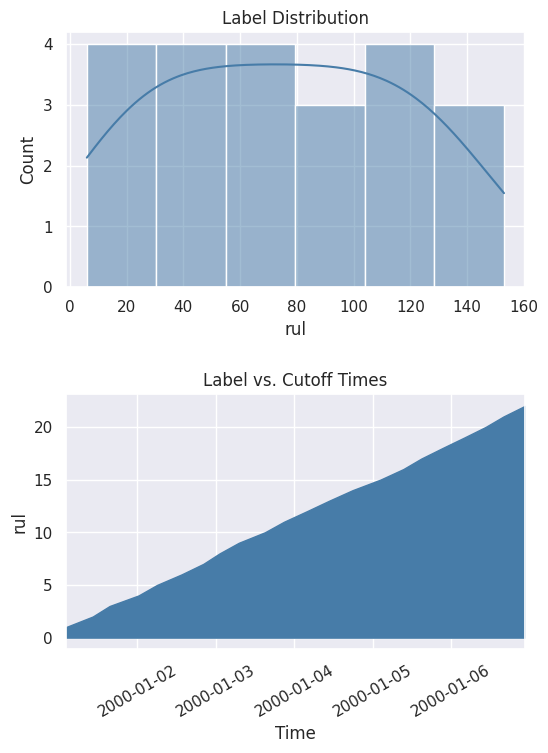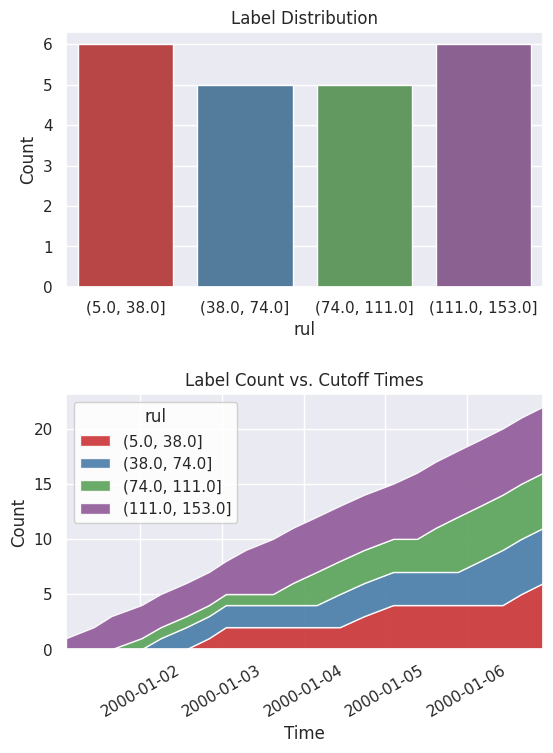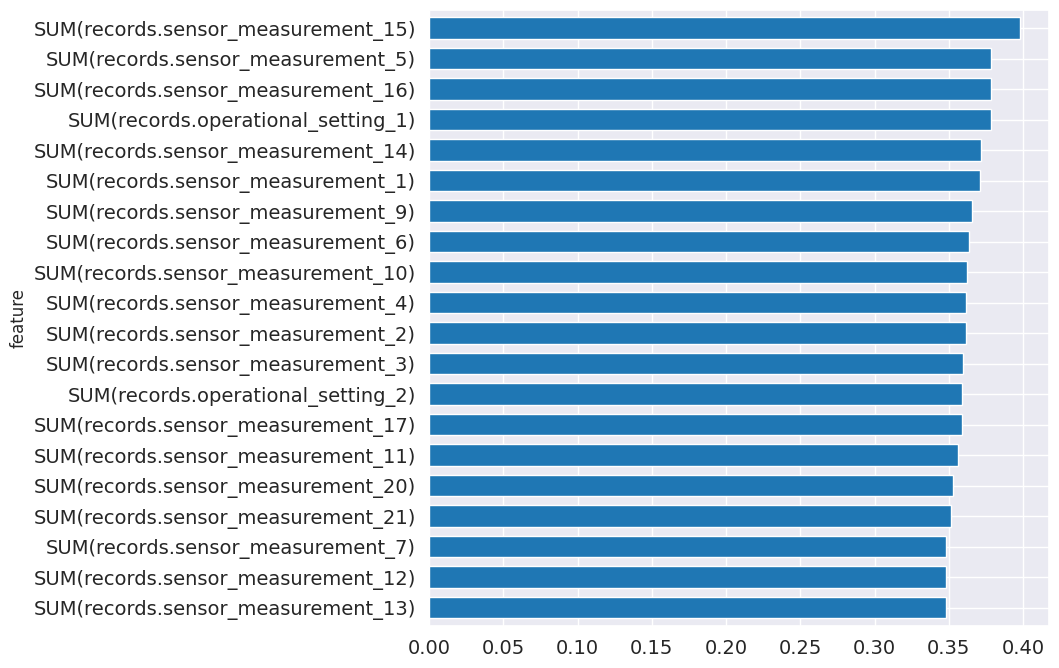In this tutorial, build a machine learning application to predict turbofan engine degradation. This application is structured into three important steps:

• Prediction Engineering

• Feature Engineering

• Machine Learning

In the first step, create new labels from the data by using Compose. In the second step, generate features for the labels by using Featuretools. In the third step, search for the best machine learning pipeline using EvalML. After working through these steps, you should understand how to build machine learning applications for real-world problems like forecasting demand.

Note: In order to run this example, you should have Featuretools 1.4.0 or newer and EvalML 0.41.0 or newer installed.

:

from demo.turbofan_degredation import load_sample
from matplotlib.pyplot import subplots
import composeml as cp
import featuretools as ft
import evalml


Use a dataset provided by NASA simulating turbofan engine degradation. In the dataset, there is data about engines that have been monitored over time. Each engine had operational settings and sensor measurements recorded over a number of cycles. The remaining useful life (RUL) is the amount of cycles an engine has left before it needs maintenance. What makes this dataset special is that the engines run all the way until failure, giving us precise RUL information for every engine at every point in time. The model you build in this tutorial predicts RUL.

:

df = load_sample()

:

engine_no time_in_cycles operational_setting_1 operational_setting_2 operational_setting_3 sensor_measurement_1 sensor_measurement_2 sensor_measurement_3 sensor_measurement_4 sensor_measurement_5 ... sensor_measurement_13 sensor_measurement_14 sensor_measurement_15 sensor_measurement_16 sensor_measurement_17 sensor_measurement_18 sensor_measurement_19 sensor_measurement_20 sensor_measurement_21 time
id
270 1 271 0.0010 0.0018 100.0 518.67 643.01 1591.11 1395.60 14.62 ... 2388.12 8157.56 8.3141 0.03 393 2388 100.0 39.26 23.5280 2000-01-02 21:00:00
401 2 81 0.0004 0.0000 100.0 518.67 642.88 1581.74 1398.46 14.62 ... 2387.98 8134.70 8.4092 0.03 392 2388 100.0 39.08 23.3215 2000-01-03 18:50:00
810 3 191 41.9983 0.8400 100.0 445.00 549.13 1352.21 1122.64 3.91 ... 2388.18 8098.80 9.2919 0.02 330 2212 100.0 10.64 6.4525 2000-01-06 15:00:00
66 1 67 35.0054 0.8400 100.0 449.44 555.43 1351.45 1109.90 5.48 ... 2387.89 8062.45 9.3215 0.02 333 2223 100.0 14.90 9.0315 2000-01-01 11:00:00
328 2 8 0.0023 0.0019 100.0 518.67 642.23 1576.51 1391.71 14.62 ... 2388.07 8135.66 8.3894 0.03 392 2388 100.0 38.95 23.4243 2000-01-03 06:40:00

5 rows × 27 columns

## Prediction Engineering¶

Which range is the RUL of a turbofan engine in?

In this prediction problem, you want to group the RUL data into ranges, then predict which range the RUL is in. You can make variations in the ranges to create different prediction problems. For example, the ranges could be manually defined (0 - 150, 150 - 300, etc.) or based on the quartiles from historical observations. Bin the RUL to make variations, helping you explore different scenarios that are crucial for making better decisions.

### Defining the Labeling Function¶

Let’s start by defining the labeling function of an engine that calculates the RUL. Given that engines run all the way until failure, the RUL is just the remaining number of observations. Our labeling function will be used by a label maker to extract the training examples.

:

def rul(ds):
return len(ds) - 1


### Representing the Prediction Problem¶

Represent the prediction problem by creating a label maker with the following parameters:

• The target_dataframe_index as the column for the engine ID, since you want to process records for each engine.

• The labeling_function as the function you defined previously.

• The time_index as the column for the event time.

:

lm = cp.LabelMaker(
target_dataframe_index='engine_no',
labeling_function=rul,
time_index='time',
)


### Finding the Training Examples¶

Run a search to get the training examples by using the following parameters:

• The records sorted by the event time, since the search expects the records to be sorted chronologically. Otherwise, an error occurs.

• num_examples_per_instance as the number of training examples to find for each engine.

• minimum_data as the amount of data to use to make features for the first training example.

• gap as the number of rows to skip between examples. This is done to cover different points in time of an engine.

You can easily tweak these parameters and run more searches for training examples as the requirements of our model change.

:

lt = lm.search(
df.sort_values('time'),
num_examples_per_instance=20,
minimum_data=5,
gap=20,
verbose=False,
)


:

engine_no time rul
0 1 2000-01-01 02:10:00 153
1 1 2000-01-01 10:20:00 133
2 1 2000-01-01 15:30:00 113
3 1 2000-01-02 00:20:00 93
4 1 2000-01-02 06:00:00 73

The output from the search is a label times table with three columns:

• The engine ID associated to the records. There can be many training examples generated from each engine.

• The event time of the engine. This is also known as a cutoff time for building features. Only data that existed beforehand is valid to use for predictions.

• The value of the RUL. This is calculated by the labeling function.

At this point, you only have continuous values of the RUL. As a helpful reference, you can print out the search settings that were used to generate these labels.

:

lt.describe()

Label Distribution
------------------
count     22.000000
mean      75.045455
std       43.795496
min        6.000000
25%       37.750000
50%       74.000000
75%      111.250000
max      153.000000

Settings
--------
gap                                  20
maximum_data                       None
minimum_data                          5
num_examples_per_instance            20
target_column                       rul
target_dataframe_index        engine_no
target_type                  continuous
window_size                        None

Transforms
----------
No transforms applied



You can also get a better look at the values by plotting the distribution and the cumulative count across time.

:

%matplotlib inline
fig, ax = subplots(nrows=2, ncols=1, figsize=(6, 8))
lt.plot.distribution(ax=ax)
lt.plot.count_by_time(ax=ax)With the continuous values, you can explore different ranges without running the search again. In this case, use quartiles to bin the values into ranges.

:

lt = lt.bin(4, quantiles=True, precision=0)


When you print out the settings again, you can now see that the description of the labels has been updated and reflects the latest changes.

:

lt.describe()

Label Distribution
------------------
(5.0, 38.0]        6
(38.0, 74.0]       5
(74.0, 111.0]      5
(111.0, 153.0]     6
Total:            22

Settings
--------
gap                                 20
maximum_data                      None
minimum_data                         5
num_examples_per_instance           20
target_column                      rul
target_dataframe_index       engine_no
target_type                   discrete
window_size                       None

Transforms
----------
1. bin
- bins:            4
- labels:       None
- precision:       0
- quantiles:    True
- right:        True



Look at the new label distribution and cumulative count across time.

:

fig, ax = subplots(nrows=2, ncols=1, figsize=(6, 8))
lt.plot.distribution(ax=ax)
lt.plot.count_by_time(ax=ax)## Feature Engineering¶

In the previous step, you generated the labels. The next step is to generate features.

### Representing the Data¶

Let’s start by representing the data with an EntitySet. That way, you can generate features based on the relational structure of the dataset. You currently have a single table of records where one engine can have many records. This one-to-many relationship can be represented by normalizing an engine dataframe. The same can be done for other one-to-many relationships. Because you want to make predictions based on the engine, you should use this engine dataframe as the target for generating features.

:

es = ft.EntitySet('observations')

dataframe=df.reset_index(),
dataframe_name='records',
index='id',
time_index='time',
)

es.normalize_dataframe(
base_dataframe_name='records',
new_dataframe_name='engines',
index='engine_no',
)

es.normalize_dataframe(
base_dataframe_name='records',
new_dataframe_name='cycles',
index='time_in_cycles',
)

es.plot()

:### Calculating the Features¶

Now you can generate features by using a method called Deep Feature Synthesis (DFS). That method automatically builds features by stacking and applying mathematical operations called primitives across relationships in an entityset. The more structured an entityset is, the better DFS can leverage the relationships to generate better features. Run DFS with these parameters:

• entityset as the entityset we structured previously.

• target_dataframe_name as the engine dataframe.

• cutoff_time as the label times that we generated previously. The label values are appended to the feature matrix.

:

fm, fd = ft.dfs(
entityset=es,
target_dataframe_name='engines',
agg_primitives=['sum'],
trans_primitives=[],
cutoff_time=lt,
cutoff_time_in_index=True,
include_cutoff_time=False,
verbose=False,
)


:

SUM(records.operational_setting_1) SUM(records.operational_setting_2) SUM(records.operational_setting_3) SUM(records.sensor_measurement_1) SUM(records.sensor_measurement_10) SUM(records.sensor_measurement_11) SUM(records.sensor_measurement_12) SUM(records.sensor_measurement_13) SUM(records.sensor_measurement_14) SUM(records.sensor_measurement_15) ... SUM(records.sensor_measurement_20) SUM(records.sensor_measurement_21) SUM(records.sensor_measurement_3) SUM(records.sensor_measurement_4) SUM(records.sensor_measurement_5) SUM(records.sensor_measurement_6) SUM(records.sensor_measurement_7) SUM(records.sensor_measurement_8) SUM(records.sensor_measurement_9) rul
engine_no time
1 2000-01-01 02:10:00 144.0091 3.1408 460.0 2320.65 5.29 207.99 1125.75 11579.85 40197.21 47.2120 ... 88.81 53.7315 6888.78 5769.91 34.97 49.96 1196.94 10949.70 42003.06 (111.0, 153.0]
2000-01-01 10:20:00 696.0567 16.5674 2340.0 11679.31 26.46 1048.19 5771.01 58258.12 201039.56 235.5492 ... 457.84 275.0327 34711.17 29110.65 178.43 255.68 6133.60 55278.36 210997.96 (111.0, 153.0]
2000-01-01 15:30:00 1074.1074 26.1125 4340.0 21363.69 49.45 1932.52 12306.22 106015.78 362843.58 414.1002 ... 961.65 577.5433 64026.68 54108.93 366.96 532.23 13073.84 101404.29 384766.02 (111.0, 153.0]
2000-01-02 00:20:00 1507.1531 36.1607 6300.0 30844.92 72.28 2799.74 18039.10 153414.42 524573.72 594.5083 ... 1409.19 845.9532 92685.14 78469.12 534.32 776.40 19160.56 146707.66 556304.58 (74.0, 111.0]
2000-01-02 06:00:00 1916.1945 46.6147 8180.0 40426.89 94.48 3658.46 23971.12 200093.76 685705.98 779.0373 ... 1872.64 1124.1869 121291.99 102704.45 712.65 1033.81 25466.22 191571.92 727679.61 (38.0, 74.0]

5 rows × 25 columns

There are two outputs from DFS: a feature matrix and feature definitions. The feature matrix is a table that contains the feature values with the corresponding labels based on the cutoff times. Feature definitions are features in a list that can be stored and reused later to calculate the same set of features on future data.

## Machine Learning¶

In the previous steps, generate the labels and features. The final step is to build the machine learning pipeline.

### Splitting the Data¶

Start by extracting the labels from the feature matrix and splitting the data into a training set and a holdout set.

:

fm.reset_index(drop=True, inplace=True)
y = fm.ww.pop('rul').cat.codes

splits = evalml.preprocessing.split_data(
X=fm,
y=y,
test_size=0.2,
random_seed=2,
problem_type='multiclass',
)

X_train, X_holdout, y_train, y_holdout = splits


### Finding the Best Model¶

Run a search on the training set to find the best machine learning model. During the search process, predictions from several different pipelines are evaluated to find the best pipeline.

:

automl = evalml.AutoMLSearch(
X_train=X_train,
y_train=y_train,
problem_type='multiclass',
objective='f1 macro',
random_seed=0,
allowed_model_families=['catboost', 'random_forest'],
max_iterations=3,
)

automl.search()

:

{1: {'Logistic Regression Classifier w/ Label Encoder + Replace Nullable Types Transformer + Imputer + Standard Scaler': '00:02',
'Random Forest Classifier w/ Label Encoder + Replace Nullable Types Transformer + Imputer': '00:01',
'Total time of batch': '00:04'}}


Once the search is complete, you can print out information about the best pipeline found, like the parameters in each component.

:

automl.best_pipeline.describe()
automl.best_pipeline.graph()

********************************************************************************************************************
* Logistic Regression Classifier w/ Label Encoder + Replace Nullable Types Transformer + Imputer + Standard Scaler *
********************************************************************************************************************

Problem Type: multiclass
Model Family: Linear
Number of features: 24

Pipeline Steps
==============
1. Label Encoder
* positive_label : None
2. Replace Nullable Types Transformer
3. Imputer
* categorical_impute_strategy : most_frequent
* numeric_impute_strategy : mean
* boolean_impute_strategy : most_frequent
* categorical_fill_value : None
* numeric_fill_value : None
* boolean_fill_value : None
4. Standard Scaler
5. Logistic Regression Classifier
* penalty : l2
* C : 1.0
* n_jobs : -1
* multi_class : auto
* solver : lbfgs

:Score the model performance by evaluating predictions on the holdout set.

:

best_pipeline = automl.best_pipeline.fit(X_train, y_train)

score = best_pipeline.score(
X=X_holdout,
y=y_holdout,
objectives=['f1 macro'],
)

dict(score)

:

{'F1 Macro': 0.7}


From the pipeline, you can see which features are most important for predictions.

:

feature_importance = best_pipeline.feature_importance
feature_importance = feature_importance.set_index('feature')['importance']
top_k = feature_importance.abs().sort_values().tail(20).index
feature_importance[top_k].plot.barh(figsize=(8, 8), fontsize=14, width=.7);### Making Predictions¶

You are ready to make predictions with our trained model. Start by calculating the same set of features by using the feature definitions. Use a cutoff time based on the latest information available in the dataset.

:

fm = ft.calculate_feature_matrix(
features=fd,
entityset=es,
cutoff_time=ft.pd.Timestamp('2001-01-08'),
cutoff_time_in_index=True,
verbose=False,
)


:

SUM(records.operational_setting_1) SUM(records.operational_setting_2) SUM(records.operational_setting_3) SUM(records.sensor_measurement_1) SUM(records.sensor_measurement_10) SUM(records.sensor_measurement_11) SUM(records.sensor_measurement_12) SUM(records.sensor_measurement_13) SUM(records.sensor_measurement_14) SUM(records.sensor_measurement_15) ... SUM(records.sensor_measurement_2) SUM(records.sensor_measurement_20) SUM(records.sensor_measurement_21) SUM(records.sensor_measurement_3) SUM(records.sensor_measurement_4) SUM(records.sensor_measurement_5) SUM(records.sensor_measurement_6) SUM(records.sensor_measurement_7) SUM(records.sensor_measurement_8) SUM(records.sensor_measurement_9)
engine_no time
1 2001-01-08 3704.4218 88.2459 15140.0 75343.52 176.13 6829.64 43725.78 372863.62 1283533.42 1459.5372 ... 92440.87 3417.83 2050.7627 225986.66 191497.63 1302.86 1884.32 46451.89 356328.97 1358639.49
2 2001-01-08 3171.3342 77.7058 13100.0 67022.44 154.92 6045.93 38813.14 327720.14 1135827.75 1317.3793 ... 82000.00 3037.99 1821.3241 200451.56 170232.49 1177.62 1696.59 41242.35 313731.51 1202449.71
3 2001-01-08 3158.3517 73.5442 12120.0 62285.84 144.47 5596.92 34594.98 305518.88 1064083.44 1229.1709 ... 76036.38 2716.73 1630.8386 185464.99 157035.05 1052.49 1509.15 36748.57 291385.49 1121106.20

3 rows × 24 columns

Now predict which one of the four ranges the RUL is in.

:

y_pred = best_pipeline.predict(fm)
y_pred = y_pred.values

prediction = fm[[]]
prediction['rul (estimate)'] = y_pred

: